login  home  contents  what's new  discussion  bug reports help  links  subscribe  changes  refresh  edit

Most introductory material about Axiom applies to FriCAS. The first chapters of FriCAS book http://fricas.github.io/book.pdf may serve as a tutorial.

## A Quick Tutorial

On this web site you can enter FriCAS commands and see the output in proper mathematical form. These commands can also be typed directly into FriCAS installed on your own computer.

You must enclose each set of FriCAS commands in a \begin{axiom} \end{axiom} section. For example:

    \begin{axiom}
[1/2, 3/4, 2/3]
\end{axiom}


When you save the changes you make to a page, FriCAS processes the contents of each section you have marked and the FriCAS output is displayed.

Try this command:

fricas
(1) -> [1/7, 3/4, 5/6]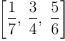(1)
Type: List(Fraction(Integer))

Please feel free to play with this in the SandBox!

Let's see if we can perform a finite window Fourier inversion.

fricas
simplify(integrate(exp(%i*_\omega*t) * exp(-%i*_\omega * _\tau), _\omega=-_\Omega .. _\Omega))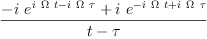(2)
Type: Expression(Complex(Integer))

Try this integrating this

fricas
2*x/sin(x)^2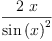(3)
Type: Expression(Integer)

fricas
integrate(%,x)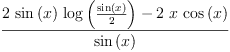(4)
Type: Union(Expression(Integer),...)

Now differentiate it

fricas
D(%,x)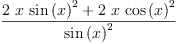(5)
Type: Expression(Integer)
fricas
simplify(%)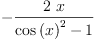(6)
Type: Expression(Integer)

Expressions (3) and (6) are really that same.

fricas
simplify(%%(3) - %%(6))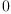(7)
Type: Expression(Integer)

fricas
D(x^x,x)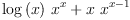(8)
Type: Expression(Integer)

See, that was easy!

Here are some more things to try.

fricas
integrate(log(sin(x)),x=0..%pi)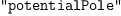(9)
Type: Union(pole: potentialPole,...)

fricas
limit(sum(1/i^2,i=1..n),n=%plusInfinity)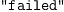(10)
Type: Union("failed",...)

Can you explain these results?

For more details see: FriCASProgramming .

## Special FriCASWiki Markup for Programming

To include Spad programs on web pages in this website click edit (or when adding comments or in bug reports) use:

       \begin{spad}
)abbrev ...
...


### Aldor

To include Aldor programs on pages in this website use:

       \begin{aldor}
...
\end{aldor}


or:

       \begin{aldor}[name]
...
\end{aldor}


### LaTeX

You can add LaTeX formatted equations to pages on this web site by:

       $$...$$


and inline symbols such as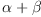are written like this: $\alpha +\beta$

To include arbitrary LaTeX on pages in this website use:

       \begin{latex}
...
\end{latex}


### Lisp

To include Lisp programs on pages in this website use:

       \begin{lisp}
...
\end{lisp}


### Boot

To include Boot programs on pages in this website use:

       \begin{boot}
...
\end{boot}


 Subject:   Be Bold !! ( 14 subscribers )